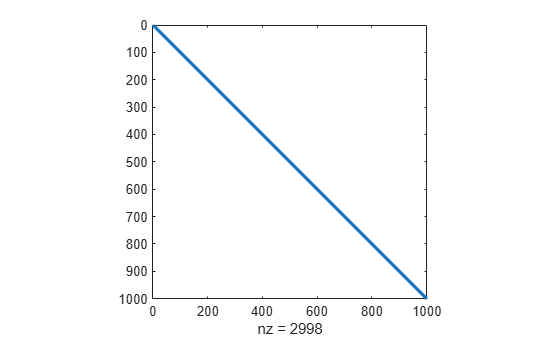# Minimization with Gradient and Hessian Sparsity Pattern

This example shows how to solve a nonlinear minimization problem with a tridiagonal Hessian matrix approximated by sparse finite differences instead of explicit computation.

The problem is to find $x$ to minimize

`$f\left(x\right)=\sum _{i=1}^{n-1}\left({\left({x}_{i}^{2}\right)}^{\left({x}_{i+1}^{2}+1\right)}+{\left({x}_{i+1}^{2}\right)}^{\left({x}_{i}^{2}+1\right)}\right),$`

where $n$ = 1000.

`n = 1000;`

To use the `trust-region` method in `fminunc`, you must compute the gradient in the objective function; it is not optional as in the `quasi-newton` method.

The helper function `brownfg` at the end of this example computes the objective function and gradient.

To allow efficient computation of the sparse finite-difference approximation of the Hessian matrix $H\left(x\right)$, the sparsity structure of $H$ must be predetermined. In this case, the structure `Hstr`, a sparse matrix, is available in the file `brownhstr.mat`. Using the `spy` command, you can see that `Hstr` is, indeed, sparse (only 2998 nonzeros).

```load brownhstr spy(Hstr)```Set the `HessPattern` option to `Hstr` using `optimoptions`. When such a large problem has obvious sparsity structure, not setting the `HessPattern` option uses a great amount of memory and computation unnecessarily, because `fminunc` attempts to use finite differencing on a full Hessian matrix of one million nonzero entries.

To use the Hessian sparsity pattern, you must use the `trust-region` algorithm of `fminunc`. This algorithm also requires you to set the `SpecifyObjectiveGradient` option to `true` using `optimoptions`.

```options = optimoptions(@fminunc,'Algorithm','trust-region',... 'SpecifyObjectiveGradient',true,'HessPattern',Hstr);```

Set the objective function to `@brownfg`. Set the initial point to –1 for odd $x$ components and +1 for even $x$ components.

```xstart = -ones(n,1); xstart(2:2:n,1) = 1; fun = @brownfg;```

Solve the problem by calling `fminunc` using the initial point `xstart` and options `options`.

`[x,fval,exitflag,output] = fminunc(fun,xstart,options);`
```Local minimum found. Optimization completed because the size of the gradient is less than the value of the optimality tolerance. ```

Examine the solution and solution process.

`disp(fval)`
``` 7.4738e-17 ```
`disp(exitflag)`
``` 1 ```
`disp(output)`
``` iterations: 7 funcCount: 8 stepsize: 0.0046 cgiterations: 7 firstorderopt: 7.9822e-10 algorithm: 'trust-region' message: 'Local minimum found....' constrviolation: [] ```

The function $f\left(x\right)$ is a sum of powers of squares and, therefore, is nonnegative. The solution `fval` is nearly zero, so it is clearly a minimum. The exit flag `1` also indicates that `fminunc` finds a solution. The `output` structure shows that `fminunc` takes only seven iterations to reach the solution.

Display the largest and smallest elements of the solution.

`disp(max(x))`
``` 1.9955e-10 ```
`disp(min(x))`
``` -1.9955e-10 ```

The solution is near the point where all elements of `x = 0`.

### Helper Function

This code creates the `brownfg` helper function.

```function [f,g] = brownfg(x) % BROWNFG Nonlinear minimization test problem % % Evaluate the function n=length(x); y=zeros(n,1); i=1:(n-1); y(i)=(x(i).^2).^(x(i+1).^2+1) + ... (x(i+1).^2).^(x(i).^2+1); f=sum(y); % Evaluate the gradient if nargout > 1 if nargout > 1 i=1:(n-1); g = zeros(n,1); g(i) = 2*(x(i+1).^2+1).*x(i).* ... ((x(i).^2).^(x(i+1).^2))+ ... 2*x(i).*((x(i+1).^2).^(x(i).^2+1)).* ... log(x(i+1).^2); g(i+1) = g(i+1) + ... 2*x(i+1).*((x(i).^2).^(x(i+1).^2+1)).* ... log(x(i).^2) + ... 2*(x(i).^2+1).*x(i+1).* ... ((x(i+1).^2).^(x(i).^2)); end end```